# Solutions of liquids in liquids

## SOLUTIONS OF LIQUIDS IN LIQUIDS

– The solutions of liquids in liquids may be divided into three classes as follows: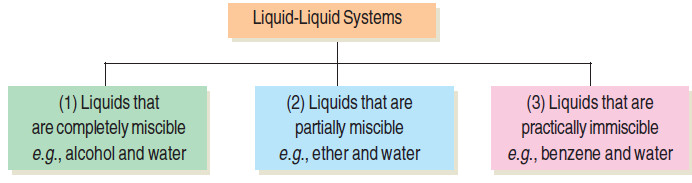– We will now proceed to consider the more important properties of these three classes of solutions.

## SOLUBILITY OF COMPLETELY MISCIBLE LIQUIDS

– Liquids like alcohol and ether mix in all proportions and in this respect they could be compared to gases.

– The properties of such solutions, however, are not strictly additive, and therefore their study has not proved of much interest.

– Generally the volume decreases on mixing but in some cases it increases. Sometimes heat is evolved when they are mixed while in others it is absorbed.

– The separation of this type of solutions can be effected by fractional distillation.

## SOLUBILITY OF PARTIALLY MISCIBLE LIQUIDS

– A large number of liquids are known which dissolve in one another only to a limited extent e.g., ether and water. Ether dissolves about 1.2% water; and water also dissolves about 6.5% ether. Since their mutual solubilities are limited, they are only partially miscible. When equal volumes of ether and water are shaken together, two layers are formed, one of a saturated solution of ether in water and the other of a saturated solution of water in ether. These two solutions are referred to as conjugate solutions.

– The effect of temperature on the mutual solubility of these mixtures of conjugate solutions is of special interest.

– We will study the effect of temperature on the composition of such mixtures with reference to three typical systems :

(1) Phenol-Water system

(2) Triethylamine-Water system

(3) Nicotine-Water system

## PHENOL – WATER SYSTEM

The curve in the following fig. represents the miscibility of phenol and water.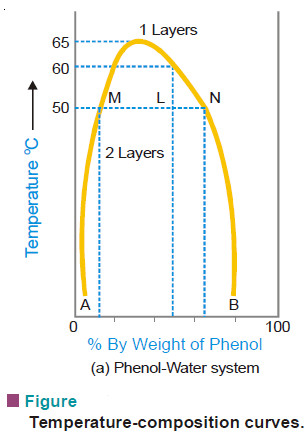– The left hand side of the parabolic curve represents one of the two conjugate solutions which depicts the percentage of phenol dissolved in water at various temperatures.

– The solubility of phenol increases with temperature.

– The right hand side of the curve represents the other conjugate solution layer that gives the percentage of water in phenol.

– The solubility of water in phenol also increase with increase of temperature.

– The two solution curves meet at the maxima on the temperature-composition curve of the system. This point here corresponds to temperature 66°C and composition of phenol as 33%.

– Thus at a certain maximum temperature the two conjugate solutions merge, become identical and only one layer results.

– The temperature at which the two conjugate solutions (or layers) merge into one another to from one layer, is called the Critical Solution Temperature (CST) or Upper Consolate Temperature.

– This is characteristic of a particular system and is influenced very much by the presence of impurities.

– The determination of critical solution temperature may, therefore, be used for testing the purity of phenol and other such substances.

– At any temperature above the critical solution temperature, phenol and water are miscible in all proportions.

– Outside the curve there is complete homogeneity of the system, i.e., one layer only exists; and under the curve there may be complete miscibility but it depends upon the composition of the mixture.

– It is clear from the previous Fig. that at a temperature below 50°C a mixture of 90% phenol and 10% water or 5% phenol and 95% water, will be completely miscible since the corresponding points do not lie under the curve.

– Two layers will always separate out below the curve and the curve gives compositions of the conjugate solutions constituting the two layers.

– At 50°C a mixture of equal proportion of phenol and water (50% each) will form two layers whose compositions are given by A and B.

– The line joining the points (M and N) corresponding to the compositions A and B is called the tie line.

– This line helps in calculating the relative amounts of the two layers, which is here given by the ratio MN/ML.

– Some other liquid pairs behaving like phenol water system are given below with their CST values and the percentage of the first component being given in bracket.

(a) Methanol-Cyclohexane (49°C ; 29)

(b) Hexane-Aniline (59.6°C ; 52)

(c) Carbon disulphide-Methanol (49.5°C, 80)

## TRIETHYLAMINE–WATER SYSTEM

The temperature-composition curve of mutual solubilities of triethylamine and water is given in the following fig.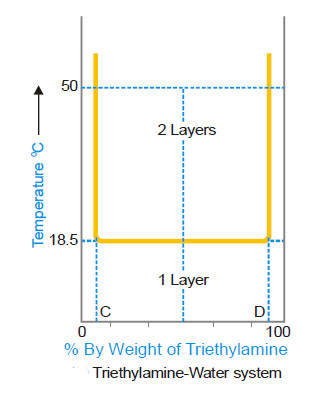– The left hand side of the curve indicates the solubility curve of triethylamine in water and the right hand side of that of water in triethylamine.

– Unlike phenol-water system, the solubilities decrease with the increase in temperature in this system.

– The two conjugate solutions mix up completely at or below 18.5°C. This temperature is also called the critical solution temperature or the lower consolate temperature.

– As in the above case, any point above the horizontal line corresponds to heterogeneity of the system (two layers) while below it is complete homogeneity (one layer).

– Thus an equi-component mixture (50–50) will be completely miscible at 10°C but at 50°C there will be separating out two layers having compositions corresponding to the points C and D.

– Common examples of this system with their lower critical solution temperatures and percentage of the first component are given below.

(a) Diethylamine-Water (43°C ; 13)

(b) 1-Methylpiperidine-Water (48°C ; 5)

## NICOTINE–WATER SYSTEM

– The behaviour of this type of system is as if it were a combination of the first two types.

– At ordinary temperature nicotine and water are completely miscible but at a higher temperature the mutual solubility decreases and as the temperature is raised further the two liquids again become miscible.

– In other words, the mutual solubility increases both on lowering as well as raising the temperature in certain ranges. Thus we have a closed solubility curve and the system has two critical-solution-temperatures, the upper 208°C and the lower 61°C.

– The effect of pressure on this system is that the lower critical temperature is raised while the upper critical temperature is lowered gradually until finally they become one. At this point the liquids are miscible at all the temperatures (see Fig).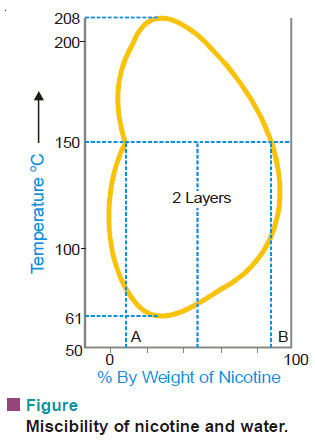Glycerine – m-Toluidine ; and Water – β-Picoline are other examples of this type.

## VAPOUR PRESSURES OF LIQUID–LIQUID SOLUTIONS

– The study of the vapour pressures of mixtures of completely miscible liquids has proved of great help in the separation of the liquids by fractional distillation.

– The vapour pressures of two liquids with varying composition have been determined at constant temperature.

– By plotting the vapour pressure against composition it has been revealed that, in general, mixtures of the miscible liquids are of three types.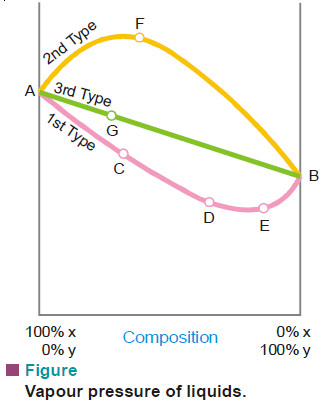### First Type of Mixtures of Miscible Liquids

– For this type of solutions the vapour pressure curve exhibits a minimum.

– If we take a mixture which has an excess of X (more volatile component), we are somewhere at C on the curve.

– When this is distilled the vapour will contain excess of X and thus the remaining mixture will get richer in Y.

– Finally we reach the point D where vapour pressure is minimum and thus boiling point is maximum. Here the mixture will distil unchanged in composition.

– Exactly similarly if we take a mixture having a greater proportion of Y (point E), on distillation Y will pass over leaving the residue richer in X till in this way the minimum point D is again reached when the mixture will distil unchanged.

– It is obvious that complete separation of this type of solutions into components is impossible.

– At best it can be resolved into one pure component and the constant boiling mixture.

– Solutions of this type which distil unchanged at a constant temperature and show a maximum boiling point are called maximum boiling point azeotropic solutions.

– The best known example of this type is presented by hydrochloric acid which forms a constant boiling mixture at 110°C and containing 20.24% of the acid.

– If a mixture of any composition is distilled, either hydrochloric acid or water will pass over, the composition will move to the point of minimum vapour pressure when it distills without any change in composition.

### Second Type of Mixtures of Miscible Liquids (Minimum boiling point azeotropic solutions)

– Here the vapour pressure curve records a maximum at F.

– At this point the mixture has the highest vapour pressure and, therefore, the lowest boiling point. Thus in this type of solutions the first fraction will consist of a constant boiling mixture with a fixed composition corresponding to the maximum point until whole of one component has been exhausted.

– After this the temperature will rise and the other component will pass over In this kind of solutions also it is not possible to effect a complete separation by fractional distillation.

– At best we can resolve it into a constant-boiling mixture and one component in the pure state.

– Ethanol and water mixtures offer a good example of this type. Ethanol-water mixture containing 95.6 per cent ethanol boils at the minimum temperature 78.13°. Thus it is very difficult to obtain pure absolute alcohol by distillation.

– This difficulty has, however, been overcome by adding benzene which form a low boiling mixture with water and on distillation it comes over leaving pure ethanol behind.

### Third Type of Mixtures of Miscible Liquids

– In this case the vapour pressures of mixtures always lie between the vapour pressures of pure components and thus the vapour-pressure composition curve is a straight line.

– Suppose we have a mixture containing excess of Y which is represented by point G on the curve. On distillation X component being more volatile will be obtained in greater proportion in the distillate and we gradually travel along the curve AB.

– The latter fractions will, of course, be poorer in X and richer in Y till we reach the 100 per cent Y-axis, when all the X will have passed over.

– By repeating the process of distillation with the fresh distillate which is now richer in X, we can get almost pure component X.

– Only in this type of solutions we can completely separate the components by fractional distillation.

– Thus methyl alcohol-water mixtures can be resolved into pure components by distillation.

– Liquid mixtures which distil with a change in composition are called zeotropic mixtures.

### Azeotropes are Mixtures and not Pure Compounds

– Although the azeotropes boil at a constant temperature and distil over without change in composition at any given pressure like a pure chemical compound, these cannot be regarded as chemical compounds.

– The reason is that when the total pressure is changed, both the boiling point and the composition of the azeotrope change whereas for a chemical compound the composition must remain constant over a range of temperature and pressures.

## THEORY OF FRACTIONAL DISTILLATION

– We have discussed above the vapourpressure composition curves for the three types of solutions from a study of which we conclude that it is only in the case of the third type that a complete separation by distillation is possible.

– However to understand the process of fractional distillation we must have an idea of the composition of the vapour phase and that of the liquid mixtures at different boiling temperatures.

– Thus for this purpose it is not the vapour-pressure composition curve but rather the temperature-composition curve that is important.

– If we plot the boiling point of liquid mixture against its composition and the composition of the vapour in contact with it, we get two separate curves for each type of solutions.

– The curves obtained for the third type are shown diagrammatically in Fig.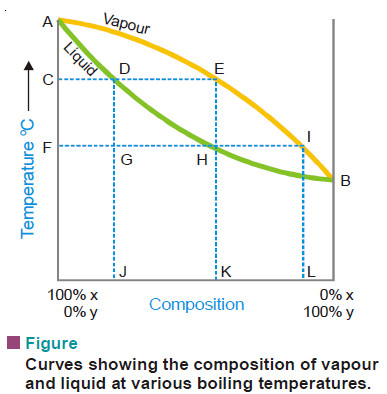– The curves AEB and ADB are the temperature composition curves for the vapour and liquid respectively.

– At any boiling temperature C the composition of liquid mixture is represented by J and that of the vapour in equilibrium by K. Obviously, the more volatile component Y is present in greater proportion in the vapour than the liquid mixture. Thus the condensed vapour or the distillate will be richer in X.

– If the distillate so obtained be now subjected to distillation, it will boil at F and the fresh distillate will have the composition L corresponding to I. Thus the proportion of Y in the second distillate is greater than in the first one.

– In this way by repeating the process of fractional distillation it is obvious that we can get almost pure Y.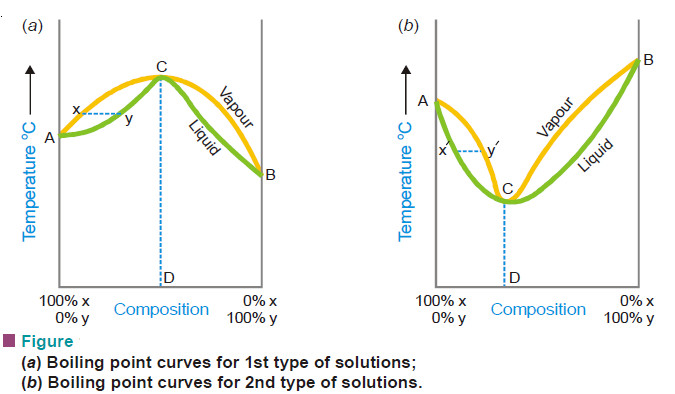– In first type of solutions (Fig. a) if we have a boiling mixture represented by Y its vapour will be poor in Y than the liquid mixture and the boiling point would gradually rise till we reach the maximum point C where the composition of liquid and vapour is the same.

– Here the distillation proceeds without change of composition. Similarly in the second type (Fig. b), if we have a boiling mixture represented by the point X’, the amount of Y in vapour is higher and gradually the boiling point falls to the minimum C’ where the vapour and the liquid mixtures have the same composition.

– At this temperature the mixture boils without any change in composition. Thus it is proved that the second and first type of solutions are not capable of being separated by fractional distillation.

– The efficiency of the process of fractional distillation is considerably enhanced by the use of the so-called Fractionating columns. These are of different designs. An effective and simple fractionating column usually employed for laboratory use consists of a long glass tube packed with glass beads (Fig. a) or specially made porcelain rings.

– The glass tube blown into bulbs at intervals may also constitute a fractionating column. For industrial purposes a fractionating tower (Fig. b) is employed.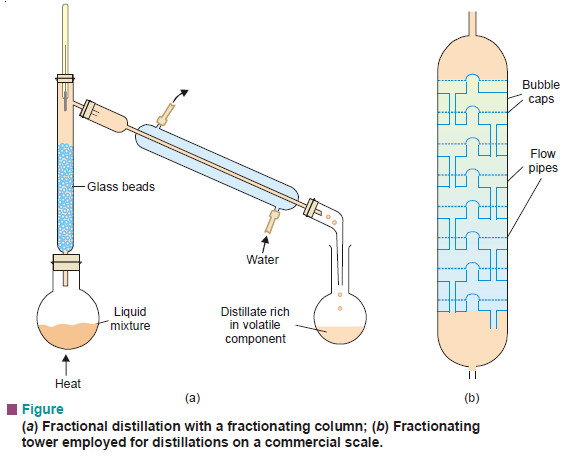– A fractionating tower is divided into several compartments by means of tray that are set one above the other. There is a hole in the centre of every tray which is covered by bubble cap.

– Each tray has an overflow pipe that joins it with the tray below by allowing the condensed liquid to flow down.

– The fractionating column or tower is fitted in the neck of the distillation flask or the still so that the vapours of the liquid being heated pass up through it.

– The temperature falls in the column as vapours pass from bottom to the top. The hot vapours that enter the column get condensed first in the lowest part of it.

– As heating is continued more vapours ascend the column and boil the liquid already condensed, giving a vapour which condenses higher up in the column.

– This liquid is heated in turn by more vapours ascending the column. Thus the liquid condensed in the lowest part is distilled on to the upper part.

– In this manner a sort of distillation and condensation goes on along the height of the column which results in the increase of the proportion of the volatile component in the outgoing vapours.

– At every point in the column there exists an equilibrium between liquid and vapour. This is established quickly by and upward flow of vapours and the downward flow of liquid, a large surface area and a slow rate of distillation.

– A simple distillation of a mixture of methanol and water and the liquid vapour equilibrium states are depicted in Fig.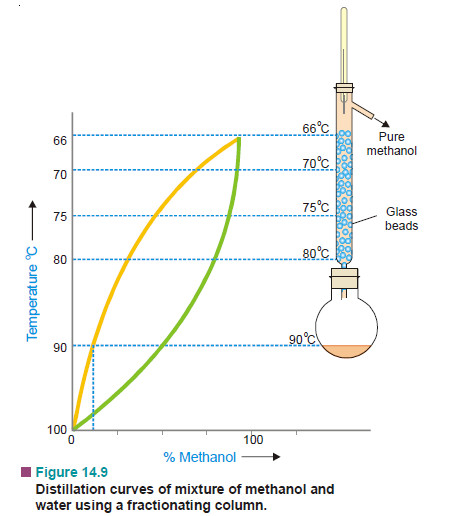– It is clear that the liquid-vapour equilibria change regularly in moving up the column. We may withdraw mixtures of varied compositions from different points on the column.

– This is done in the fractional distillation of crude oil in a refinery where different products of industrial use are conveniently separated.

## VAPOUR PRESSURE OF MIXTURES OF NON–MISCIBLE LIQUIDS

– In a mixture of non-miscible liquids each component exerts its own vapour pressure independent of others and the total vapour pressure is thus equal to the sum of individual vapour pressures of all the liquids.

– Consider two liquids in separate compartments. Each one of them would have a certain vapour pressure, say, p1 and p2. When present in a vessel with a common top, they exert their own vapour pressure independently. According to Dalton’s law of partial pressures, the total vapour pressure will be equal to the sum of their individual pressures.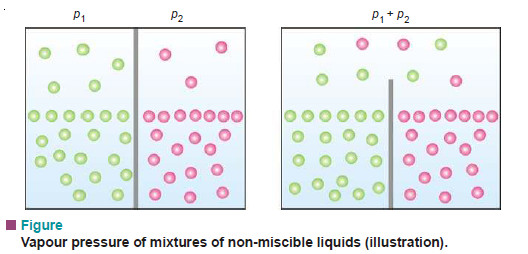– This generalisation which is the basic principle of steam distillation, has been tested experimentally in several cases. Some of the results obtained by Regnault are given below: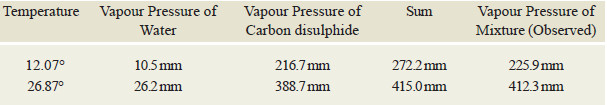– The observed vapour pressure of the mixture is a little less than the sum of the individual vapour pressures of water and carbon disulphide and that is to be expected since each liquid is slightly soluble in the other.

## STEAM DISTILLATION

– Distillation carried in a current of steam is called steam distillation. This technique is widely used for purification of organic liquids which are steam volatile and immiscible with water (e.g., aniline).

– The impure organic liquid admixed with water containing nonvolatile impurities is heated and steam passed into it.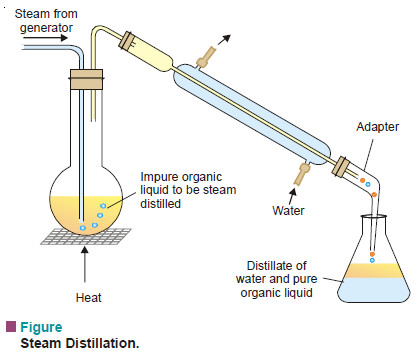– The vapour of the organic liquid and steam rising from the boiling mixture pass into the condenser.

– The distillate collected in the receiver consists of two layers, one of the pure organic liquid and the other of water.

– The pure liquid layer is removed by means of a separatory funnel and further purified.

### Theory of Steam Distillation

– The vapour pressure of a liquid rises with increase of temperature.

– When the vapour pressure equals the atmospheric pressure, the temperature recorded is the boiling point of the given liquid.

– In case of a mixture of two immiscible liquids, each component exert its own vapour pressure as if it were alone.

– The total vapour pressure over the mixture (P) is equal to the sum of the individual vapour pressures (p1, p2) at that temperature.

P = p1 + p2

– Hence the mixture will boil at a temperature when the combined vapour pressure P, equals the atmospheric pressure.

– Since P > p1 or p2, the boiling point of the mixture of two liquids will be lower than either of the pure components.

– In steam distillation the organic liquid is mixed with water (bp 100°C). Therefore the organic liquid will boil at a temperature lower than 100°C. For example, phenylamine (aniline) boils at 184°C but the steam distillation temperature of aniline is 98°C.

– Steam distillation is particularly used for the purification of an organic liquid (such as phenylamine) which decomposes at the boiling point and ordinary distillation is not possible.

### Relative Amounts of Organic Liquid and Water Distilling Over

– The number of molecules of each component in the vapour will be proportional to its vapour pressure i.e., to the vapour pressure of the pure liquid at that temperature.

Hence,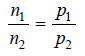(1)

where n1 and n2 are the number of moles of the two components in the vapour.

Now, from (1), we have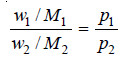(2)

where w1 and w2 are the masses of the two liquids distilling over, and M1 and M2 their molecular weights.

Since one of the two components is water (m .wt. = 18), we can write from (2)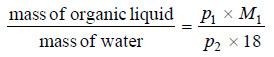– Thus, the ratio of masses of the organic liquid and water can be calculated from the given values of p1, p2 (aqueous tension) and M1, the molecular weight of the organic liquid.

## SOLVED PROBLEM

(1) At a pressure of 760 mm, a mixture of nitrobenzene (C6H5NO2) and water boils at 99°C. The vapour pressure of water at this temperature is 733 mm. Find the proportion of water and nitrobenzene in the distillate obtained by steam distillation of impure C6H5NO2.

SOLUTION

We apply the relation: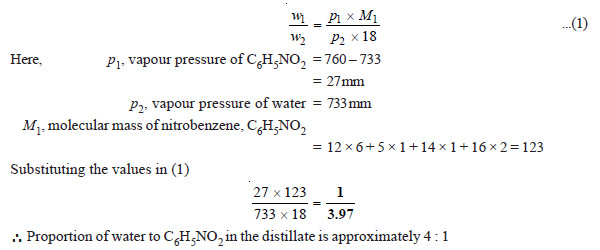(2) A mixture of water and bromobenzene (C6H5Br) distills at 95°C, and the distillate contains 1.6 times as much C6H5Br as water by mass. At 95°C the vapour pressure of water and C6H5Br are 640 mm Hg and 120 mm Hg respectively. Calculate the molecular weight of bromobenzene.

SOLUTION

We use the relation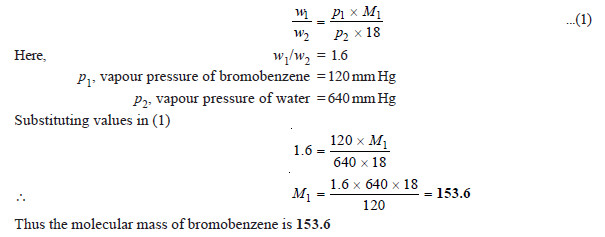Note: This method of determination of molecular weight of organic liquids is not accurate and gives only approximate value